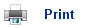# Bond Energies

• All chemical reactions involve the breaking and making of bonds. Bonds break in the reactants and then reform to make the products. The energy changes in the reactions come from the difference in energies between when the bonds are broken, and when they are made.
• Chemical bonds are electrostatic forces of attractions between atoms or ions. To break the bond you must give the elements energy until the atoms or ions are far enough apart to no longer be attracted to each other.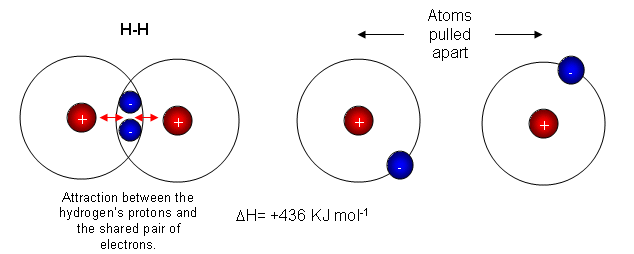• The diagram above shows two hydrogen atoms. In the first picture they are bonded together by a covalent bond. When the atoms are given energy, they pull apart from each other, eventually this pulling force overcomes the electrostatic attraction, and the bond breaks.
• The energy needed to break a bond is called the bond enthalpy or bond energy.
• The bond enthalpy of a H-H bond is 436 KJ mol-1, so it requires 436 KJ to break every H-H bond, and 436 KJ is given out for every H-H bond that is formed.
• Bond energies also tell us how strong the bond is. The higher the bond energy, the stronger the bond is.
• Double bonds have a much higher bond enthalpy than single bonds.
• In general, the higher the bond enthalpy, the shorter the bond, as the attraction is stronger.

## Measuring bond lengths

• Bond energies are very hard to measure, because there are usually many bonds in a compound. This is why energy cycles are used.

## Breaking and making bonds in a chemical reaction

• The breaking and making bonds in a reaction are responsible for the energy change. For example,

CH4 (g) + 2O2(g)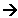CO2(g) + 2H2O (g)
• This reaction involves making and breaking bonds.
• The CH4(g) contains 4 C-H bonds, and the 2 moles of oxygen contain 1 O=O bond each. These bonds are broken and this requires energy.
• The CO2 contains 2 C=O bonds, and the 2 water molecules contain 2 O-H bonds. Energy is produced when these bonds are formed.
• The difference between these two energies is the enthalpy change.
• The energy required to break the bonds and start the reaction is called the Activation energy. Some reactions require little energy which is available from the room temperature. Other reactions require more energy, which can come from them being heated.
• It isnĺt necessary for all bonds to break before the reaction starts.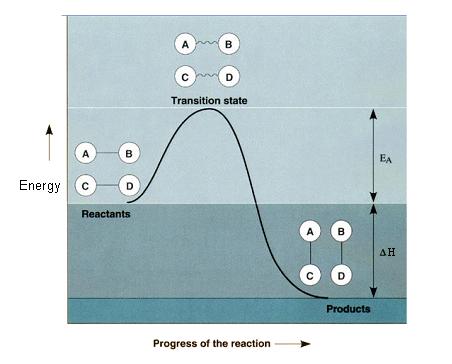• Chemists represent energy changes using an energy profile.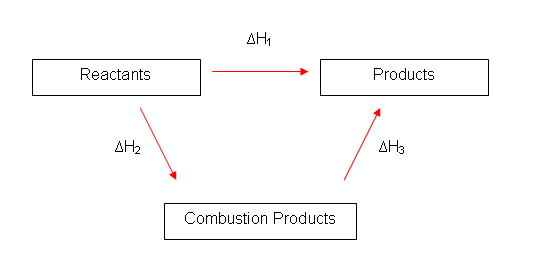• The bond-breaking and bond-making can also be represented in an energy cycle. Going through H1 is the same as going through H2 and H3.
• Bond energies do have one disadvantage, and that is that the bond enthalpy is only an average. Enthalpy changes of combustion and formation are much more accurate.
Example:

Work out the enthalpy change of combustion of Methane, using bond energies.

CH4(g) + 2O2 (g)CO2(g) + H2 O(g)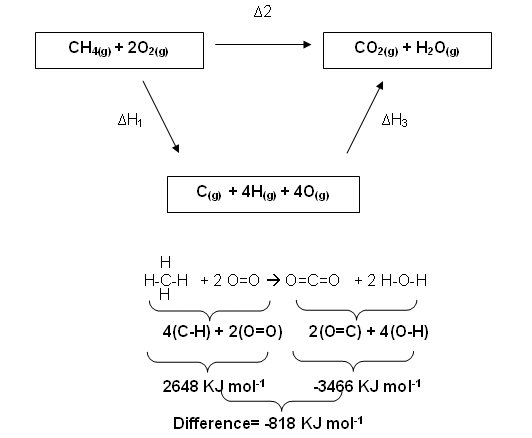• This value is different from the -890 KJ mol-1 that is should be. This is because the bond energies are only averages, and the elements are all in their gaseous states, which is not the case in standard enthalpy changes.

## Useful books for revision:

Revise AS Chemistry for Salters (Written by experienced examiners and teachers of Salter's chemistry)Revise AS Chemistry for Salters (OCR) (Salters Advanced Chemistry)Home